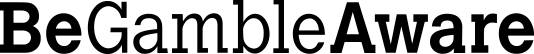# Convert Decimal Odds

Sports bettors who understand decimal odds but happen to come across fractional odds from time to time might have some trouble understanding how the two formats relate. For the benefit of those bettors we will now provide the formula for converting Fractional Odds to Decimal Odds. You will also find a Ready Reckoner table that shows the convert decimal odds for the most common fractional odds you are likely to encounter.

## Odds to Decimal

The formula for converting fractional odds to their decimal odds equivalent is actually quite simple, and can be summarised as follows:

(a / b) + 1

Where a is the left hand side of the fractional odds and b is the right hand side of the fractional odds. The formula therefore involves taking the left hand side of the fractional odds and dividing by the right hand side, then adding 1 to the result to give a decimal odds figure that includes your returned stake.

For example, odds of 11/8 would be converted as follows:

11 divided by 8 = 1.375
1.375 + 1 = 2.375
11/8 is therefore 2.375 in decimal odds format.

The same formula applies even if the left hand side of the odds is smaller than the right hand side (so-called odds-on situation). For example, odds of 8/13 would convert as follows:

8 divided by 13 = 0.615
0.615 + 1 = 1.615
8/13 is therefore 1.615 in decimal odds format.

If you want to convert fractional odds to decimal odds that don’t include a returned stake element then you should simply use the (a / b) part of the formula and refrain from adding 1 to the result.• Decimal Odds • Fractional Odds • Converting Odds • Probabilities • Ready Reckoner